Next: All-pass filters Up: Designing filters Previous: Time-varying coefficients   Contents   Index

Impulse responses of recirculating filters

In Section 7.4 we analyzed the impulse response of a recirculating comb filter, of which the one-pole low-pass filter is a special case. Figure 8.22 shows the result for two low-pass filters and one complex one-pole resonant filter. All are elementary recirculating filters as introduced in Section 8.2.3. Each is normalized to have unit maximum gain.

In the case of a low-pass filter, the impulse response gets longer (and lower) as the pole gets closer to one. Suppose the pole is at a point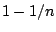(so that the cutoff frequency is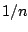radians). The normalizing factor is also. After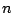points, the output diminishes by a factor ofwhereis Euler's constant, about 2.718. The filter can be said to have a settling time ofsamples. In the figure,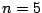for part (a) andfor part (b). In general, the settling time (in samples) is approximately one over the cutoff frequency (in angular units).The situation gets more interesting when we look at a resonant one-pole filter, that is, one whose pole lies off the real axis. In part (c) of the figure, the pole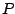has absolute value 0.9 (as in part b), but its argument is set toradians. We get the same settling time as in part (b), but the output rings at the resonant frequency (and so at a period of 10 samples in this example).

A natural question to ask is, how many periods of ringing do we get before the filter decays to strength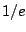? If the pole of a resonant filter has magnitudeas above, we have seen in Section 8.2.3 that the bandwidth (call it) is about, and we see here that the settling time is about. The resonant frequency (call it) is the argument of the pole, and the period in samples of the ringing is. The number of periods that make up the settling time is thus:where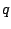is the quality of the filter, defined as the center frequency divided by bandwidth. Resonant filters are often specified in terms of the center frequency and q" in place of bandwidth.Next: All-pass filters Up: Designing filters Previous: Time-varying coefficients   Contents   Index
Miller Puckette 2006-12-30